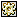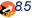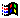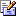Contents Articles Behaviors Books Director News Director Web Sites FAQ Games Mailing Lists News Groups Project Examples Reviews Software Tools Useful Web Sites Utilities Xtras

 Don't miss theseColour Conversions Galore!

 Compatibilities:This item has not yet been rated

Author: Chunick (website)

I have added my colour conversion handlers for anyone interested. I have not tested them all, but they are straight conversions from the information gleaned from the links which I've also provided. If you are interested in further information and other conversions or the introduction to a complicated world of accurate colour reproduction/reprsentation then the links will also be helpful in that regard. Enjoy!

 -- http://www.easyrgb.com/math.html -- http://www.brucelindbloom.com/index.html?Equations.html -- http://www.cs.rit.edu/~ncs/color/a_spaces.html -- http://www.f4.fhtw-berlin.de/~barthel/ImageJ/ColorInspector/HTMLHelp/farbraumJava.htm -- http://www.efg2.com/Lab/Graphics/Colors/index.html -- http://www.nebulus.org/tutorials/2d/photoshop/color/index.html on CMYKtoRGB (C, M, Y, K)   if C > 1 or M > 1 or Y > 1 or K > 1 then     C = C / 100.0     M = M / 100.0     Y = Y / 100.0     K = K / 100.0   end if   R = (1 - C * (1 - K) - K) * 256   G = (1 - M * (1 - K) - K) * 256   B = (1 - Y * (1 - K) - K) * 256   return color(R, G, B) end on RGBtoCMYK (R, G, B)   C = 1 - (R / 255.0)   M = 1 - (G / 255.0)   Y = 1 - (B / 255.0)   if min(C, M, Y) = 1 then return [0, 0, 0, 1]      K = min(C,M,Y)   C = (C - K) / (1 - K)   M = (M - K) / (1 - K)   Y = (Y - K) / (1 - K)   the floatPrecision = 3   return [C, M, Y, K] end on RGBtoCMY (R, G, B)   C = 1 - (R / 255.0)   M = 1 - (G / 255.0)   Y = 1 - (B / 255.0)   return [C, M, Y] end on CMYtoRGB (C, M, Y)   if C > 1 or M > 1 or Y > 1 then     C = C / 100.0     M = M / 100.0     Y = Y / 100.0     K = K / 100.0   end if   R = (1 - C) * 255   G = (1 - M) * 255   B = (1 - Y) * 255   return color(R, G, B) end on CMYtoCMYK (C, M, Y)   K = 1   if C < K then K = C   if M < K then K = M   if Y < K then K = Y      C = (C - K) / (1 - K)   M = (M - K) / (1 - K)   Y = (Y - K) / (1 - K)      return [C, M, Y, K]    end on CMYKtoCMY (C, M, Y, K)   C = ( C * ( 1 - K ) + K )   M = ( M * ( 1 - K ) + K )   Y = ( Y * ( 1 - K ) + K )   return [C, M, Y] end on RGBtoHex(R, G, B)   return color(R, G, B).hexString() end on HextoRGB(aHex)   return rgb(aHex) end -- 0, 3, 6, 9, C, F -- 0%, 20%, 40%, 60%, 80%, 100% on RGBtoWebSafe(R, G, B)   webSafe = [0, 51, 102, 153, 204, 255]   webSafe.sort()   R1 = webSafe[webSafe.findPosNear(R)]   if R < max(0, R1 - 25.5) then R1 = webSafe[max(1,webSafe.getPos(R) - 1)]   G1 = webSafe[webSafe.findPosNear(G)]   if G < max(0, G1 - 25.5) then G1 = webSafe[max(1,webSafe.getPos(G) - 1)]   B1 = webSafe[webSafe.findPosNear(B)]   if B < max(0, B1 - 25.5) then B1 = webSafe[max(1,webSafe.getPos(B) - 1)]   aColor = rgb(R1, G1, B1)   return aColor.hexString() end on WebSafetoRGB(aHex)   webSafe = ["0": 0, "3": 51, "6": 102, "9": 153, "C": 204, "F": 255]   R = webSafe.getProp(aHex.char)   G = webSafe.getProp(aHex.char)   B = webSafe.getProp(aHex.char)   return color(R, G, B) end on HextoWebSafe(aHex)   aColor = rgb(aHex)   webSafe = [0, 51, 102, 153, 204, 255]   webSafe.sort()   R = webSafe[webSafe.findPosNear(aColor.red)]   if aColor.red < max(0, R - 25.5) then R = webSafe[max(1,webSafe.getPos(R) - 1)]   G = webSafe[webSafe.findPosNear(aColor.green)]   if aColor.green < max(0, G - 25.5) then G = webSafe[max(1,webSafe.getPos(G) - 1)]   B = webSafe[webSafe.findPosNear(aColor.blue)]   if aColor.blue < max(0, B - 25.5) then B = webSafe[max(1,webSafe.getPos(B) - 1)]   aColor = rgb(R, G, B)   return aColor.hexString() end -- Colour Conversion Algorithms -- http://www.easyrgb.com/math.html -- http://www.cs.rit.edu/~ncs/color/t_convert.html -- RGB to Hue, Saturation, Value on RGBtoHSV(R, G, B)      R = R / 255.0   G = G / 255.0   B = B / 255.0   aMin = min(R, G, B)   aMax = max(R, G, B)   delta = aMax - aMin   V = aMax      if aMax = 0 then     S = 0     H = 0   else     S = delta / float(aMax)     if R = aMax then       H = (G - B) / float(delta)     else if G = aMax then       H = 2 + (B - R) / float(delta)     else       H = 4 + (R - G) / float(delta)     end if     H = 60 * H     if H < 0 then H = H + 360   end if      return [H, S, V] end on RGBtoHSB (R, G, B)   return RGBtoHSV(R, G, B) end on HSVtoRGB(H, S, V)   if S = 0 then     R = V     G = V     B = V   end if   H = H / 60.0   i = integer(H - 0.499999)   f = H - i   p = V * (1 - S)   q = V * (1 - S * f)   t = V * (1 - S * (1 - f))   Case i of     0:       R = V       G = t       B = p     1:       R = p       G = v       B = p     2:       R = p       G = V       B = t     3:       R = p       G = q       B = V     4:       R = t       G = p       B = V     otherwise       R = V       G = p       B = q   end Case      return color(R * 255, G * 255, B * 255)    end on HSBtoRGB (H, S, B)   return HSVtoRGB(H, S, B) end -- RGB to Hue, Saturation, Lightness on RGBtoHSL (R, G, B)      R = R / 255.0   G = G / 255.0   B = B / 255.0   aMin = min(R, G, B)   aMax = max(R, G, B)   delta = aMax - aMin   L = (aMax + aMin ) / 2      if aMax = 0 then     S = 0     H = 0   else     if  L < 0.5 then       S = delta / ( aMax + aMin )     else                 S = delta / ( 2 - aMax - aMin )     end if     if R = aMax then       H = (G - B) / float(delta)     else if G = aMax then       H = 2 + (B - R) / float(delta)     else       H = 4 + (R - G) / float(delta)     end if     H = 60 * H     if H < 0 then H = H + 360   end if      return [H, S, L] end -- this function calls another function: HuetoRGB( v1, v2, vH ) on HSLtoRGB (H, S, L)      if S = 0 then     -- HSL values 0-360, 0-1, 0-1     R = L * 255                      --RGB results = 0 - 255     G = L * 255     B = L * 255        else          if L < 0.5 then       temp2 = L * (1.0 + S)     else       temp2 = (L + S) - (S * L)     end if          if H > 1 then H = H / 360.0        -- HSL values = 0 - 1     temp1 = (2.0 * L) - temp2          R = 255 * HuetoRGB( temp1, temp2, H + ( 1.0 / 3.0 ) )     G = 255 * HuetoRGB( temp1, temp2, H )     B = 255 * HuetoRGB( temp1, temp2, H - ( 1.0 / 3.0 ) )        end if      return color(R, G, B)    end on HuetoRGB( v1, v2, vH )                  if vH < 0 then vH = vH + 1   if vH > 1 then vH = vH - 1   if ( 6 * vH ) < 1 then     put (v1 + ( v2 - v1 ) * 6 * vH)     return (v1 + ( v2 - v1 ) * 6 * vH)   end if   if ( 2 * vH ) < 1 then     put v2     return v2   end if   if ( 3 * vH ) < 2 then     put (v1 + ( v2 - v1 ) * ( ( 2.0 / 3.0 ) - vH ) * 6)     return (v1 + ( v2 - v1 ) * ( ( 2.0 / 3.0 ) - vH ) * 6)   end if   return v1    end on RGBtoXYZ (R, G, B)   R = ( R / 255.0 )        --Where R = 0 - 255   G = ( G / 255.0 )        --Where G = 0 - 255   B = ( B / 255.0 )        --Where B = 0 - 255      if R > 0.04045 then     R = power((( R + 0.055 ) / 1.055 ), 2.4)   else                       R = R / 12.92   end if   if G > 0.04045 then     G = power((( G + 0.055 ) / 1.055 ), 2.4)   else                       G = G / 12.92   end if   if B > 0.04045 then     B = power((( B + 0.055 ) / 1.055 ), 2.4)   else                       B = B / 12.92   end if      R = R * 100   G = G * 100   B = B * 100      --Observer. = 2°, Illuminant = D65   X = (0.412453 * R) + (0.357580 * G) + (0.180423 * B)   Y = (0.212671 * R) + (0.715160 * G) + (0.072169 * B)   Z = (0.019334 * R) + (0.119193 * G) + (0.950227 * B)      return [X, Y, Z] end on XYZtoRGB (X, Y, Z)      X = X / 100.0       --Where X = 0 -  95.047   Y = Y / 100.0       --Where Y = 0 - 100.000   Z = Z / 100.0       --Where Z = 0 - 108.883      --Observer = 2°, Illuminant = D65   R = (3.240479 * X) + (-1.537150 * Y) + (-0.498535 * Z)   G = (-0.969256 * X) + (1.875992 * Y) + (0.041556 * Z)   B = (0.055648 * X) + (-0.204043 * Y) + (1.057311 * Z)      if ( R > 0.0031308 ) then     R = 1.055 * power(R, 1 / 2.4) - 0.055   else                         R = 12.92 * R   end if   if ( G > 0.0031308 ) then     G = 1.055 * power(G, 1 / 2.4) - 0.055   else                         G = 12.92 * G   end if   if ( B > 0.0031308 ) then     B = 1.055 * power(B, 1 / 2.4) - 0.055   else                         B = 12.92 * B   end if      R = R * 255   G = G * 255   B = B * 255      return color(R, G, B) end on XYZtoCIELab (X, Y, Z)   -- Observer= 2°, Illuminant= D65   X = X / 95.047          --refX =  95.047     Y = Y / 100.000         --refY = 100.000   Z = Z / 108.883         --refZ = 108.883      if ( X > 0.008856 ) then     X = power(X, 1.0/3.0)   else                         X = ( 7.787 * X ) + ( 16.0 / 116.0 )   end if      if ( Y > 0.008856 ) then     Y = power(Y, 1.0/3.0)   else                         Y = ( 7.787 * Y ) + ( 16.0 / 116.0 )   end if      if ( Z > 0.008856 ) then     Z = power(Z, 1.0/3.0)   else                         Z = ( 7.787 * Z ) + ( 16.0 / 116.0 )   end if      L = ( 116 * Y ) - 16   a = 500 * ( X - Y )   b = 200 * ( Y - Z )      return [L, a, b]    end on CIELabtoXYZ (L, a, b)      Y = ( L + 16 ) / 116.0   X = ( a / 500.0 )+ Y   Z = Y - ( b / 200.0 )      if ( power(Y, 3) > 0.008856 ) then     Y = power( Y, 3)   else                           Y = ( Y - (16.0 / 116.0 )) / 7.787   end if      if ( power(X, 3) > 0.008856 ) then     X = power(X, 3)   else                           X = ( X - (16.0 / 116.0) ) / 7.787   end if      if ( power(Z, 3) > 0.008856 ) then     Z = power(Z, 3)   else                           Z = ( Z - (16.0 / 116.0) ) / 7.787   end if         X = 95.047 * X      --refX =  95.047  Observer= 2°, Illuminant= D65   Y = 100.000 * Y     --refY = 100.000   Z = 108.883 * Z     --refZ = 108.883      return [X, Y, Z] end on XYZtoCIELuv (X, Y, Z)      U = ( 4 * X ) / ( X + ( 15 * Y ) + ( 3 * Z ) )   V = ( 9 * Y ) / ( X + ( 15 * Y ) + ( 3 * Z ) )      Y = Y / 100.0   if ( Y > 0.008856 ) then     Y = power(Y,  1/3.0)   else                         Y = ( 7.787 * Y ) + ( 16 / 116.0 )   end if         refX =  95.047        --Observer= 2°, Illuminant= D65   refY = 100.000   refZ = 108.883      refU = ( 4 * refX ) / ( refX + ( 15 * refY ) + ( 3 * refZ ) )   refV = ( 9 * refY ) / ( refX + ( 15 * refY ) + ( 3 * refZ ) )      L = ( 116 * Y ) - 16   U = 13 * L * ( U - refU )   V = 13 * L * ( V - refV )      return [L, U, V]    end on CIELuvtoXYZ (L, U, V)      Y = ( L + 16 ) / 116.0   if ( power(Y, 3) > 0.008856 ) then     Y = power(Y, 3)   else                           Y = ( Y - (16 / 116.0) ) / 7.787   end if      refX =  95.047      --Observer= 2°, Illuminant= D65   refY = 100.000   refZ = 108.883      refU = ( 4 * refX ) / ( refX + ( 15 * refY ) + ( 3 * refZ ) )   refV = ( 9 * refY ) / ( refX + ( 15 * refY ) + ( 3 * refZ ) )      U = U / ( 13 * L ) + refU   V = U / ( 13 * L ) + refV      Y = Y * 100   X =  - ( 9 * Y * U ) / ( ( U - 4 ) * V - U * V )   Z = ( 9 * Y - ( 15 * V * Y ) - ( V * X ) ) / ( 3 * V )      return [X, Y, Z] end on XYZtoHunterLab (X, Y, Z)   HL = 10 * sqrt( Y )   Ha = 17.5 * ( ( ( 1.02 * X ) - Y ) / sqrt( Y ) )   Hb = 7 * ( ( Y - ( 0.847 * Z ) ) / sqrt( Y ) )   return [HL, Ha, Hb] end on HunterLabtoXYZ (HL, Ha, Hb)   Y = HL / 10   X = Ha / 17.5 * HL / 10   Z = Hb / 7 * HL / 10      Y = power(Y,2)   X = ( X + Y ) / 1.02   Z = -( Z - Y ) / 0.847      return [X, Y, Z] end --Where X = 0 -  95.047       Observer. = 2°, Illuminant = D65 --Where Y = 0 - 100.000 --Where Z = 0 - 108.883 on XYZtoYxy (X, Y, Z)   Y = Y   xx = X / ( X + Y + Z )   yy = Y / ( X + Y + Z )   return [Y, xx, yy]   end --Where Y = 0 - 100 --Where xx = 0 - 1 --Where yy = 0 - 1 on YxytoXYZ (Y, xx, yy)   X = xx * ( Y / yy )   Y = Y   Z = ( 1 - xx - yy ) * ( Y / yy )   return [X, Y, Z]   end -- The YIQ system is the colour primary system -- adopted by NTSC for colour television broadcasting on RGBtoYIQ (R, G, B)   Y = (0.299 * R) + (0.587 * G) + (0.114 * B)   I = (0.596 * R) + (-0.275 * G) + (-0.321 * B)   Q = (0.212 * R) + (-0.523 * G) + (0.311 * B)   return [Y, I, Q] end on YIQtoRGB (Y, I, Q)   R = (1.0 * Y) + (0.956 * I) + (0.621 * Q)   G = (1.0 * Y) + (-0.272 * I) + (-0.647 * Q)   B = (1.0 * Y) + (-1.105 * I) + (1.702 * Q)   return color(R, G, B) end -- YUV is like YIQ, except that it is the PAL/European standard on RGBtoYUV (R, G, B)   Y = (0.299 * R) + (0.587 * G) + (0.114 * B)   U = (-0.147 * R) + (-0.289 * G) + (0.437 * B)   V = (0.615 * R) + (-0.515 * G) + (-0.100 * B)   return [Y, U, V] end on YUVtoRGB (Y, U, V)   R = (1.0 * Y) + (0.0 * U) + (1.140 * V)   G = (1.0 * Y) + (-0.394 * U) + (-0.581 * V)   B = (1.0 * Y) + (2.028 * U) + (0.0 * V)   return color(R, G, B) end -- ****************************************************************** -- Sub Functions -- ****************************************************************** on CIELabtoRGB (L, a, b)   XYZ = CIELabToXYZ(L, a, b)   return XYZtoRGB(XYZ, XYZ, XYZ) end on RGBtoCIELab (R, G, B)   XYZ = RGBtoXYZ(R, G, B)   return XYZtoCIELab (XYZ, XYZ, XYZ) end -- ********************************************************************************* -- XYZ (Tristimulus) Reference values of a perfect reflecting diffuser -- ********************************************************************************* -- Observer            2° (CIE 1931)                    10° (CIE 1964) --Illuminant           X2        Y2        Z2        X10        Y10         Z10 -- --A (Tungsten)      109.850     100.0    35.585    111.144      100.0      35.200 --C                  98.074     100.0   118.232     97.285      100.0     116.145 --D50                96.422     100.0    82.521     96.720      100.0      81.427 --D55                95.682     100.0    92.149     95.799      100.0      90.926 --D65 (Daylight)     95.047     100.0   108.883     94.811      100.0     107.304 --D75                94.972     100.0   122.638     94.416      100.0     120.641 --D93 (CRT monitor) --F2 (Fluorescent)   99.187     100.0    67.395    103.280      100.0      69.026 --F7                 95.044     100.0   108.755     95.792      100.0     107.687 --F11               100.966     100.0    64.370    103.866      100.0      65.620 --Temperature       x         y      Dir y/x --(Kelvin) --2000           0.52669   0.41331   1.33101 --2105           0.51541   0.41465   1.39021 --2222           0.50338   0.41525   1.45962 --2353           0.49059   0.41498   1.54240 --2500           0.47701   0.41368   1.64291 --2677           0.463     0.41121   1.76811 % error in table , estimated values --2857           0.446     0.40742   1.92863 --3077           0.43156   0.40216   2.14300 --3333           0.41502   0.39535   2.44455 --3636           0.39792   0.38690   2.90309 --4000           0.38045   0.37676   3.68730 --4444           0.36276   0.36496   5.34398 --5000           0.34510   0.35162  11.17883 --5714           0.32775   0.33690 -39.34888 --6667           0.31101   0.32116  -6.18336 --8000           0.29518   0.30477  -3.08425 --10000          0.28063   0.28828  -1.93507 -- ************************************************************ -- The below are from: -- http://www.srgb.com/hpsrgbprof/sld001.htm -- however, I do not know if the math is correct -- ************************************************************ on XYZD65toXYZD50 (X65, Y65, Z65)   X50 = (1.0479 * X65) + (.0229 * Y65) + (-0.0502 * Z65)   Y50 = (.0296 * X65) + (.9904 * Y65) + (-0.0171 * Z65)   Z50 = (-0.0092 * X65) + (.0151 * Y65) + (.7519 * Z65)   return [X50, Y50, Z50] end -- 2.2 Gamma on XYZD50toRGB (X, Y, Z)   R = (.4361 * X) + (.2664 * Y) + (.1431 * Z)   G = (.2225 * X) + (.7169 * Y) + (.0606 * Z)   B = (.0139 * X) + (.0971 * Y) + (.7141 * Z)   return color(R, G, B) end --on XYZtoRGB (X, Y, Z) --  R = (.8951 * X) + (.2664 * Y) + (-0.1614 * Z) --  G = (-0.7502 * X) + (1.7135 * Y) + (.0367 * Z) --  B = (.0389 * X) + (.0685 * Y) + (1.0296 * Z) --  return color(R, G, B) --end -- Gamma Equation -- For each R, G, B value: -- if RGB <= 0.03928 -- RGB' = RGB/12.92 -- else -- RGB' = power((0.55 + RGB)/1.055), 2.4) -- end if -- Note: RGB normalized to 0.0 to 0.1 --on ceil myNum -- --return integer(myNum + 0.499999999999999) -- --end -- --on floor myNum -- --return integer(myNum - 0.499999999999999) -- --end --ITU-R BT.709 Primaries and white point D65 . Valid for sRGB. on sRGBtoXYZ (R, G, B)   R = R/255.0   G = G/255.0   B = B/255.0   X = (0.4124 * R) + (0.3576 * G) + (0.1805 * B)   Y = (0.2126 * R) + (0.7152 * G) + (0.0722 * B)   Z = (0.0193 * R) + (0.1192 * G) + (0.9505 * B)      return [X * 100, Y * 100, Z * 100]    end on XYZtosRGB (X, Y, Z)      X = X/100.0   Y = Y/100.0   Z = Z/100.0      R = (3.2410 * X) + (-1.5374 * Y) + ( -0.4986 * Z)   G = (-0.9692 * X) + ( 1.8760 * Y) + ( 0.0416 * Z)   B = (0.0556 * X) + ( -0.2040 * Y) + ( 1.0570 * Z)      return color(R * 255, G * 255, B * 255)    end --Basic matrix shells --  X = (0 * R) + (0 * G) + (0 * B) --  Y = (0 * R) + (0 * G) + (0 * B) --  Z = (0 * R) + (0 * G) + (0 * B) --  R = (0 * X) + (0 * Y) + (0 * Z) --  G = (0 * X) + (0 * Y) + (0 * Z) --  B = (0 * X) + (0 * Y) + (0 * Z) --The conversion for D65 RGB to D65 XYZ uses the matrix on page 14, ITU-R BT.709 Primaries. --D65 XYZ means XYZ without changing the illuminant. -- XYZD65 -> RGBD65 -- X = 0.4124 0.3576 0.1805 R -- Y = 0.2126 0.7152 0.0722 G -- Z = 0.0193 0.1192 0.9505 B on XYZ2RGB (X, Y, Z)   X = X/100.0   Y = Y/100.0   Z = Z/100.0      R = (3.240479 * X) + (-1.537150 * Y) + (-0.498535 * Z)   G = (-0.969256 * X) + (1.875992 * Y) + (0.041556 * Z)   B = (0.055648 * X) + (-0.204043 * Y) + (1.057311 * Z)      return color(R * 255, G * 255, B * 255) end on RGB2XYZ (R, G, B)   R = R/255.0   G = G/255.0   B = B/255.0      X = (0.412453 * R) + (0.357580 * G) + (0.180423 * B)   Y = (0.212671 * R) + (0.715160 * G) + (0.072169 * B)   Z = (0.019334 * R) + (0.119193 * G) + (0.950227 * B)      return [X * 100, Y * 100, Z * 100] end --The conversion for D65 RGB to D50 XYZ applies additionally (by multiplication) the Bradford --correction, which takes the adaptation of the eyes into account. This correction is an improved --alternative to the Von Kries correction . --Monitors are assumed D65, but for printed paper the standard illuminant is D50. Therefore --this transformation is recommended if the data are used for printing: -- XYZD50 -> RGBD65 -- X = 0.4361 0.3851 0.1431 R -- Y = 0.2225 0.7169 0.0606 G -- Z = 0.0139 0.0971 0.7141 B

 Contact MMI 36 South Court Sq Suite 300 Newnan, GA 30263 USASend e-mail